• Index
•
•

## Infinite Slope

The following problem for verification is a fictional case intended to compare MacSlope results with the closed form solutions for the infinite slope case. It is a 4H:1V (14 deg) slope of soil overlying an impenetrable region at 5m depth. Three cases are compared below.

The MacSlope results are calculated with the GLE method and using a specified search, with a 5m deep tension crack, to model the vertical end slices needed for the infinite slope formulation. The specified surface intersects one homogenous material, so any interslice forces at the end slices cancel each other. The end points of the specified surface do not have to be exactly on the slope surface, as long as they intersect it.

### Case 1 (c' = 0, Φ = 28o), dry

The factor of safety for a dry infinite slope in purely frictional material is given by: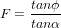For our slope,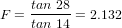The MacSlope result for this case is shown below. The factor of safety is 2.127.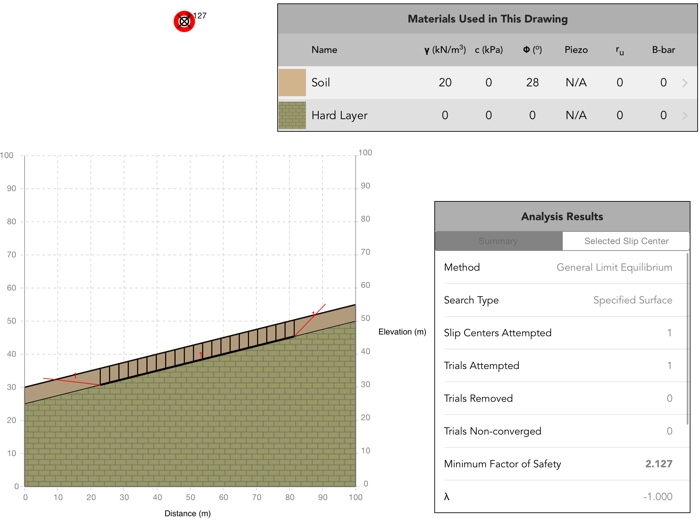### Case 2 (c' = 0, Φ = 28o), wet

The factor of safety for an infinite slope in purely frictional material, and ground water at the surface with horizontal seepage is given by,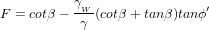For our slope,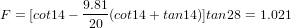The MacSlope result for this case is shown below. The factor of safety is 1.018.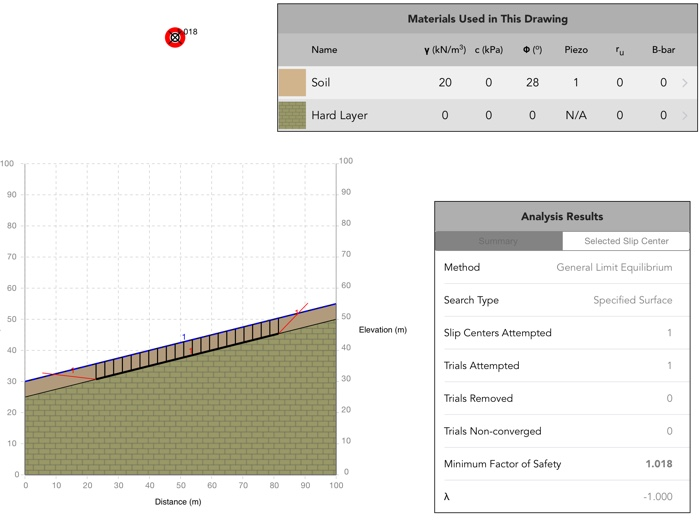### Case 3 (c' = 50 kPa, Φ = 28o), wet

The factor of safety for an infinite slope with cohesion and friction, with ground water at the surface with horizontal seepage is given by,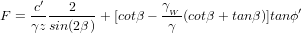For our slope,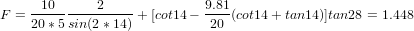The MacSlope result for this case is shown below. The factor of safety is 1.443.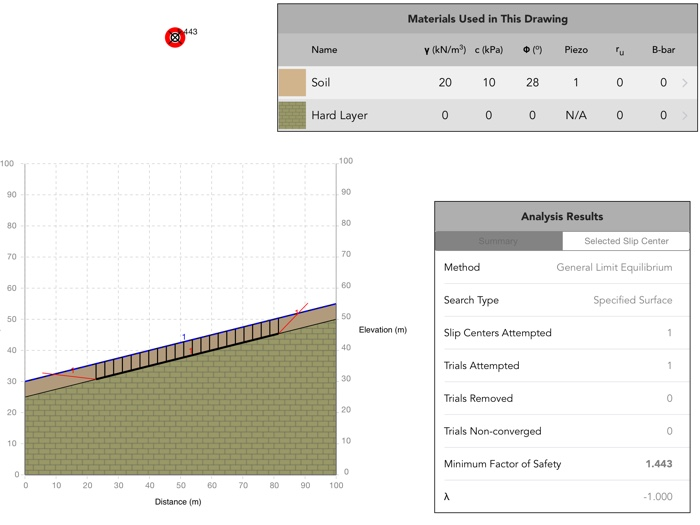Download the MacSlope file with the analysis results for this verification example here.